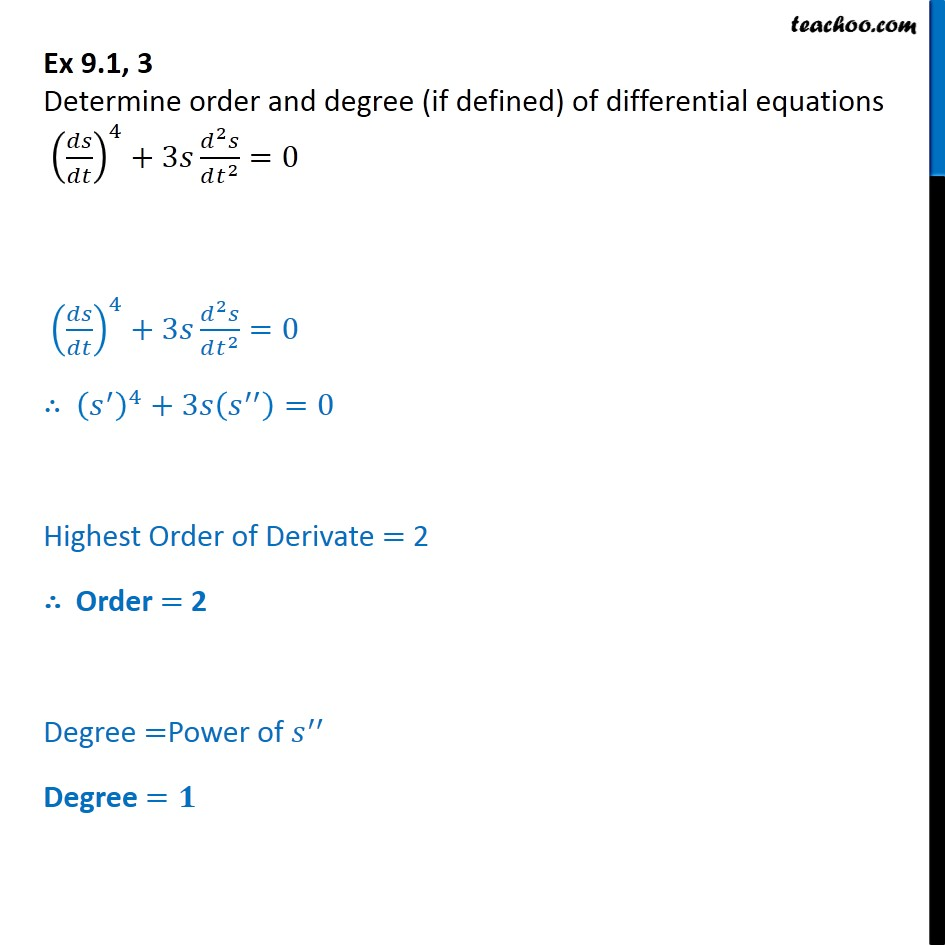Order and Degree

Chapter 9 Class 12 Differential Equations
Concept wiseIntroducing your new favourite teacher - Teachoo Black, at only ₹83 per month

### Transcript

Ex 9.1, 3 Determine order and degree (if defined) of differential equations (ds/dt)4 + 3s d2s/dt2 = 0 (s')4 + 3s (s'') = 0 Highest Order of Derivate = 2 Order = 2 Degree =Power of s Degree =